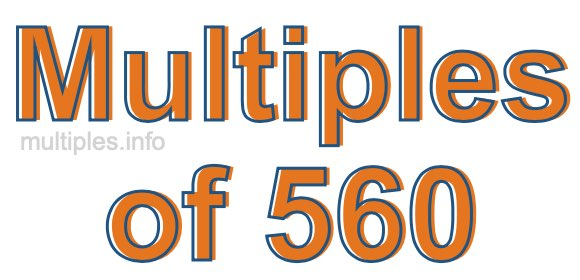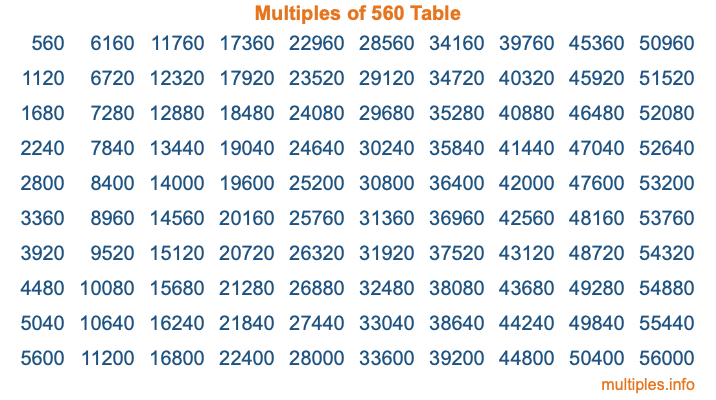Multiples of 560Welcome to the Multiples of 560 page. Here we will first teach you everything you will ever need to know about the multiples of 560, and then give you a study guide summary of everything we taught you to make sure you remember it all. Use this page to look up facts and learn information about the multiples of 560. This page will make you a multiples of five hundred sixty expert!

Definition of Multiples of 560
Multiples of 560 are all the numbers that when divided by 560 equal an integer. Each of the multiples of 560 are called a multiple. A multiple of 560 is created by multiplying 560 by an integer.

Therefore, to create a list of multiples of 560, you start with 1 multiplied by 560, then 2 multiplied by 560, then 3 multiplied by 560, and so on for as long as you want. Thus, the list of the first five multiples of 560 is 560, 1120, 1680, 2240, and 2800. To see a larger list of multiples of 560, see the printable image of Multiples of 560 further down on this page. We also have a category where you can choose any nth multiple of 560.

Multiples of 560 Checker
The Multiples of 560 Checker below checks to see if any number of your choice is a multiple of 560. In other words, it checks to see if there is any number (integer) that when multiplied by 560 will equal your number. To do that, we divide your number by 560. If the the quotient is an integer, then your number is a multiple of 560.

Is  a multiple of 560?

Least Common Multiple of 560 and ...
A Least Common Multiple (LCM) is the lowest multiple that two or more numbers have in common. This is also called the smallest common multiple or lowest common multiple and is useful to know when you are adding our subtracting fractions. Enter one or more numbers below (560 is already entered) to find the LCM.

Check out our LCM Calculator if you need more details about the Least Common Multiple or if you need the LCM for different numbers for adding and subtraction fractions.

nth Multiple of 560
As we stated above, 560 is the first multiple of 560, 1120 is the second multiple of 560, 1680 is the third multiple of 560, and so on. Enter a number below to find the nth multiple of 560.

th multiple of 560

Multiples of 560 vs Factors of 560
560 is a multiple of 560 and a factor of 560, but that is where the similarities end. All postive multiples of 560 are 560 or greater than 560. All positive factors of 560 are 560 or less than 560.

Below is the beginning list of multiples of 560 and the factors of 560 so you can compare:

Multiples of 560: 560, 1120, 1680, 2240, 2800, etc.

Factors of 560: 1, 2, 4, 5, 7, 8, 10, 14, 16, 20, 28, 35, 40, 56, 70, 80, 112, 140, 280, 560

As you can see, the multiples of 560 are all the numbers that you can divide by 560 to get a whole number. The factors of 560, on the other hand, are all the whole numbers that you can multiply by another whole number to get 560.

It's also interesting to note that if a number (x) is a factor of 560, then 560 will also be a multiple of that number (x).

Multiples of 560 vs Divisors of 560
The divisors of 560 are all the integers that 560 can be divided by evenly. Below is a list of the divisors of 560.

Divisors of 560: 1, 2, 4, 5, 7, 8, 10, 14, 16, 20, 28, 35, 40, 56, 70, 80, 112, 140, 280, 560

The interesting thing to note here is that if you take any multiple of 560 and divide it by a divisor of 560, you will see that the quotient is an integer.

Multiples of 560 Table
Below is an image of the first 100 multiples of 560 in a table. The table is in chronological order, column by column. The first column has the first ten multiples of 560, the second column has the next ten multiples of 560, and so on.The Multiples of 560 Table is also referred to as the 560 Times Table or Times Table of 560. You are welcome to print out our table for your studies.

Negative Multiples of 560
Although not often discussed or needed in math, it is worth mentioning that you can make a list of negative multiples of 560 by multiplying 560 by -1, then by -2, then by -3, and so on, to get the following list of negative multiples of 560:

-560, -1120, -1680, -2240, -2800, etc.

Multiples of 560 Summary
Below is a summary of important Multiples of 560 facts that we have discussed on this page. To retain the knowledge on this page, we recommend that you read through the summary and explain to yourself or a study partner why they hold true.

There are an infinite number of multiples of 560.

A multiple of 560 divided by 560 will equal a whole number.

560 divided by a factor of 560 equals a divisor of 560.

The nth multiple of 560 is n times 560.

The largest factor of 560 is equal to the first positive multiple of 560.

560 is a multiple of every factor of 560.

560 is a multiple of 560.

A multiple of 560 divided by a divisor of 560 equals an integer.

560 divided by a divisor of 560 equals a factor of 560.

Any integer times 560 will equal a multiple of 560.

Multiples of a Number
Here you can get the multiples of another number, all with the same attention to detail as we did for multiples of 560 on this page.

Multiples of
Multiples of 561
Did you find our page about multiples of five hundred sixty educational? Do you want more knowledge? Check out the multiples of the next number on our list!